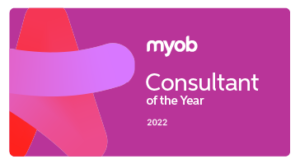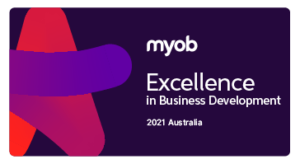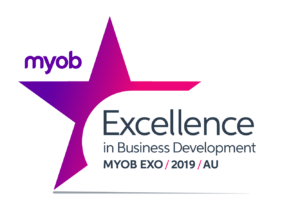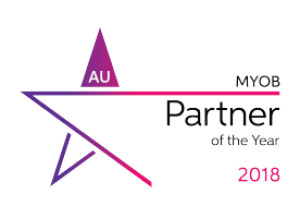# Fixed Assets in MYOB Advanced

MYOB Advanced Tips and Tricks #5

## Two new calculation methods are now available for the Australian jurisdiction:

### Let us illustrate with an Prime Cost asset example:

It is also now possible to define the percentage calculation of depreciation on the following levels per:

#### Fixed Asset (individually)

Furthermore, with the new depreciation methods, the following attributes are improvements on the old behaviour:

• Depreciation amounts are calculated on a per-day basis, which means that the calculation for a 31-day month is slightly more than for a month with 30 or fewer days.

Let us illustrate this with an example: An Asset with Acquisition Cost of \$12000 was purchased and Placed in Service on 31.03.2020 with a useful life of 1 year.
For each period of an asset’s useful life, depreciation is calculated based on the following formula.

D = Depr. Base * (Percent per Year / 100) * (N / 365)

where:

• N: The exact number of days the asset is held in the fiscal period. The number of days held is calculated as follows for the listed periods:
• The first depreciation period that is specified for the book (Depr. From Period): Last day of the period – Placed-in-Service Date + 1
• The disposal period: Disposal Date – First date of the period + 1
• All other periods: Length of the period in days

In our example, the formula calculates the following depreciation for the first three months of assets life:

• March 2020 Depr Base 12000*(100%/100)*(1 day/365)=32.88
• April 2020 Depr Base 12000*(100%/100)*(30/365)=986.30
• May 2020 Depr Base 12000*(100%/100)*(31/365)=1019.88

The same calculated depreciation amounts can be seen on the Depreciation History tab of the asset:

The pre-2020 versions Straight-Line Depreciation Calculation Method calculates the monthly depreciation to be \$1000 for all full months regardless of if the month was 28, 29, 30 or 31 days as seen below:

#### The calculation for depreciation per asset is done by changing the Depreciation percentage value

Therefore, the Fixed Asset class can then be used on a Fixed Asset, with the percentage value defaulting based on what the Fixed Asset class has set. This value can change on an asset-by-asset basis, or a book-by-book basis for each asset.

MYOB is constantly looking to improve the user experience and the software itself within MYOB Advanced. Thus, the latest release, 2020.1, introduces Employee Self Service features, and more extensive features to the Payroll module. For more information about the new Employer Self-Service Portal, read here (link to our new article).

## Interested in other ways to optimise MYOB Advanced?

Check out our handy MYOB Advanced Tips and Tricks blogs here:

We hope these tips and tricks were helpful to you! If you have any questions about Fixed Assets in MYOB Advanced, our friendly team is here to help. Call us on 1300 857 464 (AU) or 0800 436 774 (NZ), or send us an email.

Alternatively, for a comprehensive overview of all of our best MYOB Advanced ‘hacks’ in one place, you can download our PDF from the panel on the right – The Ultimate Compilations of Tips and Tricks!